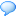Oct 27th

3

## Relative time in PHP with flexible detail level3 Comments

This post describes how you can display relative or nicer time values like 10 days, 4 hours and 6 minutes ago instead of a date like 26 October, 2009 6:45 PM. The function is flexible enough to take a parameter for the details level you desire – for example, in the above example, the detail level was 3. So, it displays days, hours and minutes. If it was 4, it would have displayed seconds too. If we were using an older date, detail level of 3 might have displayed years, months and weeks.

Here’s the code:

 `01` `/*`
 `02` ` ``* Takes a unix timestamp and returns a relative time string such as "3 minutes ago",`
 `03` ` ``*   "2 months from now", "1 year ago", etc`
 `04` ` ``* The detailLevel parameter indicates the amount of detail. The examples above are`
 `05` ` ``* with detail level 1. With detail level 2, the output might be like "3 minutes 20`
 `06` ` ``*   seconds ago", "2 years 1 month from now", etc.`
 `07` ` ``* With detail level 3, the output might be like "5 hours 3 minutes 20 seconds ago",`
 `08` ` ``*   "2 years 1 month 2 weeks from now", etc.`
 `09` ` ``*/`
 `10` `function` `nicetime(``\$timestamp``, ``\$detailLevel` `= 1) {`
 `11`
 `12` `    ``\$periods` `= ``array``(``"second"``, ``"minute"``, ``"hour"``, ``"day"``, ``"week"``, ``"month"``, ``"year"``, ``"decade"``);`
 `13` `    ``\$lengths` `= ``array``(``"60"``, ``"60"``, ``"24"``, ``"7"``, ``"4.35"``, ``"12"``, ``"10"``);`
 `14`
 `15` `    ``\$now` `= time();`
 `16`
 `17` `    ``// check validity of date`
 `18` `    ``if``(``empty``(``\$timestamp``)) {`
 `19` `        ``return` `"Unknown time"``;`
 `20` `    ``}`
 `21`
 `22` `    ``// is it future date or past date`
 `23` `    ``if``(``\$now` `> ``\$timestamp``) {`
 `24` `        ``\$difference` `= ``\$now` `- ``\$timestamp``;`
 `25` `        ``\$tense` `= ``"ago"``;`
 `26`
 `27` `    ``} ``else` `{`
 `28` `        ``\$difference` `= ``\$timestamp` `- ``\$now``;`
 `29` `        ``\$tense` `= ``"from now"``;`
 `30` `    ``}`
 `31`
 `32` `    ``if` `(``\$difference` `== 0) {`
 `33` `        ``return` `"1 second ago"``;`
 `34` `    ``}`
 `35`
 `36` `    ``\$remainders` `= ``array``();`
 `37`
 `38` `    ``for``(``\$j` `= 0; ``\$j` `< ``count``(``\$lengths``); ``\$j``++) {`
 `39` `        ``\$remainders``[``\$j``] = ``floor``(``fmod``(``\$difference``, ``\$lengths``[``\$j``]));`
 `40` `        ``\$difference` `= ``floor``(``\$difference` `/ ``\$lengths``[``\$j``]);`
 `41` `    ``}`
 `42`
 `43` `    ``\$difference` `= ``round``(``\$difference``);`
 `44`
 `45` `    ``\$remainders``[] = ``\$difference``;`
 `46`
 `47` `    ``\$string` `= ``""``;`
 `48`
 `49` `    ``for` `(``\$i` `= ``count``(``\$remainders``) - 1; ``\$i` `>= 0; ``\$i``--) {`
 `50` `        ``if` `(``\$remainders``[``\$i``]) {`
 `51` `            ``\$string` `.= ``\$remainders``[``\$i``] . ``" "` `. ``\$periods``[``\$i``];`
 `52`
 `53` `            ``if``(``\$remainders``[``\$i``] != 1) {`
 `54` `                ``\$string` `.= ``"s"``;`
 `55` `            ``}`
 `56`
 `57` `            ``\$string` `.= ``" "``;`
 `58`
 `59` `            ``\$detailLevel``--;`
 `60`
 `61` `            ``if` `(``\$detailLevel` `<= 0) {`
 `62` `                ``break``;`
 `63` `            ``}`
 `64` `        ``}`
 `65` `    ``}`
 `66`
 `67` `    ``return` `\$string` `. ``\$tense``;`
 `68`
 `69` `}`

And some examples:

 `1` `echo` `nicetime(time());`

The result would be “1 second ago”

 `1` `echo` `nicetime(time() - 839283);`

The result would be “1 week ago”

 `1` `echo` `echo nicetime(time() - 8392783);`

The result would be “3 months ago”

 `1` `echo` `echo nicetime(time() - 8392783, 3);`

The result would be “3 months 1 week 6 days ago”

 `1` `echo` `echo nicetime(time() - 83592783, 3);`

The result would be “2 years 7 months 2 weeks ago”

 `1` `echo` `echo nicetime(time() - 83592783, 5);`

The result would be “2 years 7 months 2 weeks 1 day 12 hours ago”

 `1` `echo` `echo nicetime(time() + 90532, 5);`

The result would be “1 day 1 hour 8 minutes 52 seconds from now”

### 3 Responses to “Relative time in PHP with flexible detail level”

1.php develoepr says:

I’m a little unsure on the accuracy of the function, it’s good for a basis but due to the varying amount of days in a month, 4.35 weeks is not accurate for calculating months. for example, this function would trip up on february.

And that isn’t even counting leap years

The rest is great but i feel the month’s part needs work

A good basis though

•Ankur Motreja says:

Yes, that part isn’t 100% accurate.
As you mentioned, getting it to work in all cases will require a lot of code.
Considering that the function is used to take an exact time and make it an approximate time, I think that might be unnecessary extra work for most use cases.

If anyone has the time to correct this part of the code, please do share your code :)

Ankur

2.Levi says:

I like this code!

I don’t think its a big problem that its not 100% accurate, if you are fetching users with it, nobody would care about it…
For me, it was a perfect start, just what i was looking for! If sometime i would need better accuration, i will integrate it, and send the code for you.

Thanks a lot!